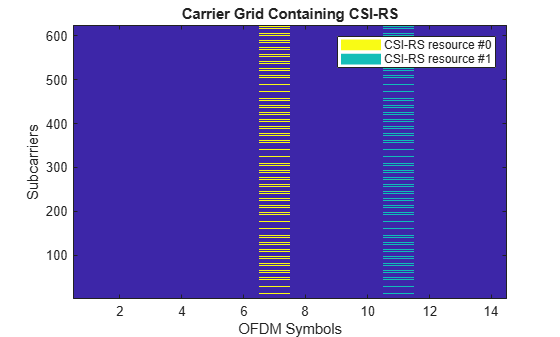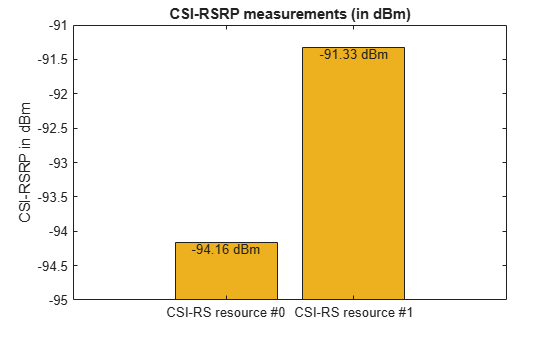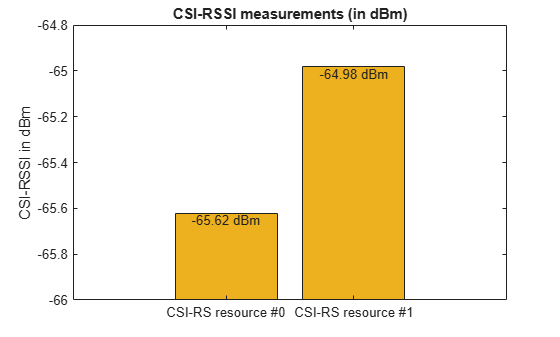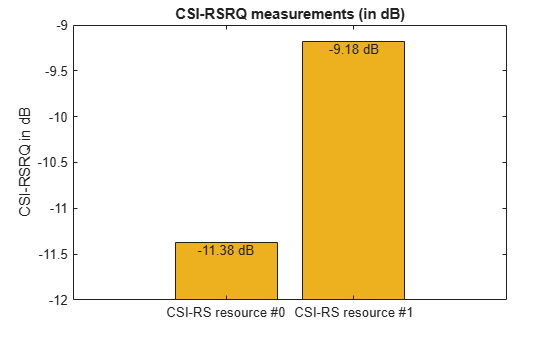Main Content

# 5G NR CSI-RS Measurements

This example shows the measurement procedure of CSI-RSRP, CSI-RSSI, and CSI-RSRQ for the test environment, as described in TS 38.133 Annex A.4.6.3.3, by using the channel state information reference signal from 5G Toolbox™.

### Introduction

In NR 5G, the three types of CSI-RS based reference signal measurements, as defined in TS 38.215 Sections 5.1.2 and 5.1.4, includes:

• CSI-RSRP (CSI reference signal received power): CSI-RSRP is defined as the linear average over the power contributions of the resource elements of the antenna ports, which carry CSI-RS configured for RSRP measurements. This measurement is performed across N number of resource blocks (measurement bandwidth). For this measurement, CSI-RS transmitted on antenna port(s) 3000 or 3000 and 3001 is used.

• CSI-RSSI (CSI received signal strength indicator): CSI-RSSI is defined as the linear average of the total received power observed only in the OFDM symbols, in which CSI-RS is present. This measurement is also performed across N number of resource blocks (measurement bandwidth). CSI-RSSI includes the power from sources, such as co-channel serving and non-serving cells, adjacent channel interference, and thermal noise. For this measurement, CSI-RS transmitted on antenna port 3000 is used.

• CSI-RSRQ (CSI reference signal received quality): CSI-RSRQ is defined as, $\mathit{N}*\frac{\mathrm{CSI}_\mathrm{RSRP}}{\mathrm{CSI}_\mathrm{RSSI}}$.

The purposes of these measurements include:

• Cell selection and reselection

• Mobility and handover management

• Beam management (beam adjustment and beam recovery)

This example configures only the CSI-RS from the test environment.

### Initialize Configuration Objects

Carrier configuration

Create carrier configuration object occupying 10 MHz bandwidth with 15 kHz subcarrier spacing as per configuration 1 in TS 38.133 Table A.4.6.3.3.1-1.

```carrier = nrCarrierConfig; carrier.NSlot = 1; carrier.NSizeGrid = 52;```

CSI-RS configuration

As per the test environment TS 38.133 Table A.4.6.3.3.2-1, user equipment (UE) is configured with one CSI-RS resource set (CSI-RS 1.2 FDD), consisting of 2 CSI-RS resources.

```csirs = nrCSIRSConfig; % CSI-RS resource #0 #1 csirs.CSIRSType = {'nzp', 'nzp'}; csirs.CSIRSPeriod = {[10 1], [10 1]}; csirs.RowNumber = [1 1]; % Single port (3000) CSI-RS resources csirs.Density = {'three', 'three'}; csirs.SymbolLocations = {6, 10}; csirs.SubcarrierLocations = {0, 0}; csirs.NumRB = [52, 52]; % Measurement bandwidth in terms of number of resource blocks```

### Generate CSI-RS Symbols and Indices

Generate CSI-RS symbols and indices for the specified carrier and CSI-RS configuration parameters with the output resource format as 'cell'. This output resource format provides a way to identify the outputs uniquely for each CSI-RS resource in a resource set. You can also apply different power levels to each CSI-RS resource.

```ind = nrCSIRSIndices(carrier,csirs,'OutputResourceFormat','cell'); sym = nrCSIRS(carrier,csirs,'OutputResourceFormat','cell');```

### Signal and Noise Power Setup

Set up the signal and noise powers as described in TS 38.133 Table A.4.6.3.3.2-2. As per note 2 in TS 38.133 Table A.4.6.3.3.2-2, interference from other cells and noise from other sources is modeled as additive white Gaussian noise (AWGN) of appropriate power `Noc`.

```SINRdB0 = 0; % For CSI-RS #0 SINRdB1 = 3; % For CSI-RS #1 NocdBm = -94.65; NocdB = NocdBm - 30; Noc = 10^(NocdB/10);```

Calculate the power scaling of CSI-RS resources by using SINR values.

```% Power scaling of CSI-RS resource #0 SINR0 = 10^(SINRdB0/10); % linear Es/Noc Es0 = SINR0*Noc; % Power scaling of CSI-RS resource #1 SINR1 = 10^(SINRdB1/10); % linear Es/Noc Es1 = SINR1*Noc;```

### Initialize the Carrier Resource Grid and Map CSI-RS Symbols to the Grid

Initialize the carrier resource grid for one slot.

```ports = max(csirs.NumCSIRSPorts); % Number of antenna ports txGrid = nrResourceGrid(carrier,ports);```

Apply the power scaling values to the CSI-RS resources and map them onto the grid.

```txGrid(ind{1}) = sqrt(Es0)*sym{1}; txGrid(ind{2}) = sqrt(Es1)*sym{2}; % Plot the carrier grid for two CSI-RS resources plotGrid(size(txGrid),ind)```### Perform OFDM modulation

Perform OFDM modulation to generate the time-domain waveform.

`[txWaveform,ofdmInfo] = nrOFDMModulate(carrier,txGrid);`

### Add AWGN to the Transmitted Waveform and Perform OFDM Demodulation

Consider the propagation condition as AWGN, as specified in TS 38.133 Table A.4.6.3.3.2-1.

```% Generate the noise rng('default'); % Set RNG state for repeatability N0 = sqrt(Noc/(2*double(ofdmInfo.Nfft))); noise = N0*complex(randn(size(txWaveform)),randn(size(txWaveform))); % Add AWGN to the transmitted waveform rxWaveform = txWaveform + noise;```

Perform OFDM demodulation on the received time-domain waveform to get the received resource element array.

`rxGrid = nrOFDMDemodulate(carrier,rxWaveform);`

### Perform CSI-RSRP, CSI-RSSI, and CSI-RSRQ Measurements

Finally, perform the CSI-RSRP, CSI-RSSI, and CSI-RSRQ measurements on the CSI-RS resources present in the received grid, by using the helper file hCSIRSMeasurements.

`meas = hCSIRSMeasurements(carrier,csirs,rxGrid)`
```meas = struct with fields: RSRPPerAntennaPerResource: [3.8372e-13 7.3692e-13] RSSIPerAntennaPerResource: [2.7403e-10 3.1752e-10] RSRQPerAntennaPerResource: [0.0728 0.1207] RSRP: [3.8372e-13 7.3692e-13] RSSI: [2.7403e-10 3.1752e-10] RSRQ: [0.0728 0.1207] RSRPdBm: [-94.1599 -91.3258] RSSIdBm: [-65.6220 -64.9823] RSRQdB: [-11.3779 -9.1834] ```
```% Plot RSRPdBm, RSSIdBm and RSRQdB measurements for all CSI-RS resources hPlotCSIRSMeasurements(meas)```You can compare the measured CSI-RSRP values of two CSI-RS resources represented by the output field `RSRPdBm` to the standard specified values given in TS 38.133 Table A.4.6.3.3.2-2.

### Local Functions

```function plotGrid(gridSize,csirsInd) % plotGrid(GRIDSIZE,CSIRSIND) plots the carrier grid of size GRIDSIZE % by populating the grid with CSI-RS symbols of multiple resources % indicated by a cell array of CSI-RS indices CSIRSIND. figure() cmap = colormap(gcf); % Considering the following values for two CSI-RS resources and they need % to be updated based on the number of CSI-RS resources names = {'CSI-RS resource #0','CSI-RS resource #1'}; chpval = {20,2}; chpscale = 0.25*length(cmap); % Scaling factor tempGrid = zeros(gridSize); tempGrid(csirsInd{1}) = chpval{1}; tempGrid(csirsInd{2}) = chpval{2}; image(chpscale*tempGrid(:,:,1)); % Multiplied with scaling factor for better visualization axis xy; clevels = chpscale*[chpval{:}]; N = length(clevels); L = line(ones(N),ones(N),'LineWidth',8); % Generate lines % Index the color map and associate the selected colors with the lines set(L,{'color'},mat2cell(cmap( min(1+clevels,length(cmap) ),:),ones(1,N),3)); % Set the colors according to cmap % Create legend legend(names{:}); title('Carrier Grid Containing CSI-RS') xlabel('OFDM Symbols'); ylabel('Subcarriers'); end```

 3GPP TS 38.133. “NR; Requirements for support of radio resource management.” 3rd Generation Partnership Project; Technical Specification Group Radio Access Network.

 3GPP TS 38.215. “NR; Physical layer measurements.” 3rd Generation Partnership Project; Technical Specification Group Radio Access Network.

Download ebook